Next: Simple harmonic motion Up: Newtonian mechanics Previous: Non-isolated systems

# Motion in one-dimensional potential

As a simple illustration of the application of Newton's laws of motion, consider a point particle of mass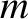moving in the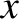-direction, say, under the action of some-directed force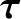. Suppose thatis a conservative force, such as gravity. In this case, according to Equation (2.19), we can write(2.45)

where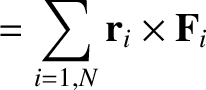is the potential energy of the particle at position.

Let the curvetake the form shown in Figure 2.4. For instance, this curve might represent the gravitational potential energy of a cyclist freewheeling in a hilly region. Observe that we have set the potential energy at infinity to zero (which we are generally free to do, because potential energy is undefined to an arbitrary additive constant). This is a fairly common convention. What can we deduce about the motion of the particle in this potential?

Well, we know that the total energy,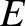--which is the sum of the kinetic energy,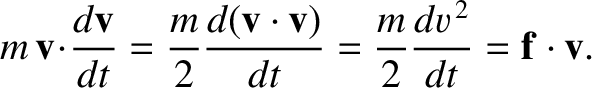, and the potential energy,--is a constant of the motion. [See Equation (2.22).] Hence, we can write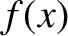(2.46)

However, we also know that a kinetic energy can never be negative, because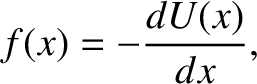, and neithernor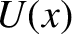can be negative. Hence, the preceding expression tells us that the particle's motion is restricted to the region (or regions) in which the potential energy curvefalls below the value. This idea is illustrated in Figure 2.4. Suppose that the total energy of the system is. It is clear, from the figure, that the particle is trapped inside one or other of the two dips in the potential; these dips are generally referred to as potential wells. Suppose that we now raise the energy to. In this case, the particle is free to enter or leave each of the potential wells, but its motion is still bounded to some extent, because it clearly cannot move off to infinity. Finally, let us raise the energy to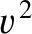. Now the particle is unbounded; that is, it can move off to infinity. In conservative systems in which it makes sense to adopt the convention that the potential energy at infinity is zero, bounded systems are characterized by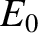, whereas unbounded systems are characterized by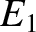.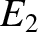The preceding discussion suggests that the motion of a particle moving in a potential generally becomes less bounded as the total energyof the system increases. Conversely, we would expect the motion to become more bounded asdecreases. In fact, if the energy becomes sufficiently small then it appears likely that the system will settle down in some equilibrium state in which the particle remains stationary. Let us try to identify any prospective equilibrium states in Figure 2.4. If the particle remains stationary then it must be subject to zero force (otherwise, it would accelerate). Hence, according to Equation (2.45), an equilibrium state is characterized by(2.47)

In other words, an equilibrium state corresponds to either a maximum or a minimum of the potential energy curve,. It can be seen that thecurve shown in Figure 2.4 has three associated equilibrium states located at,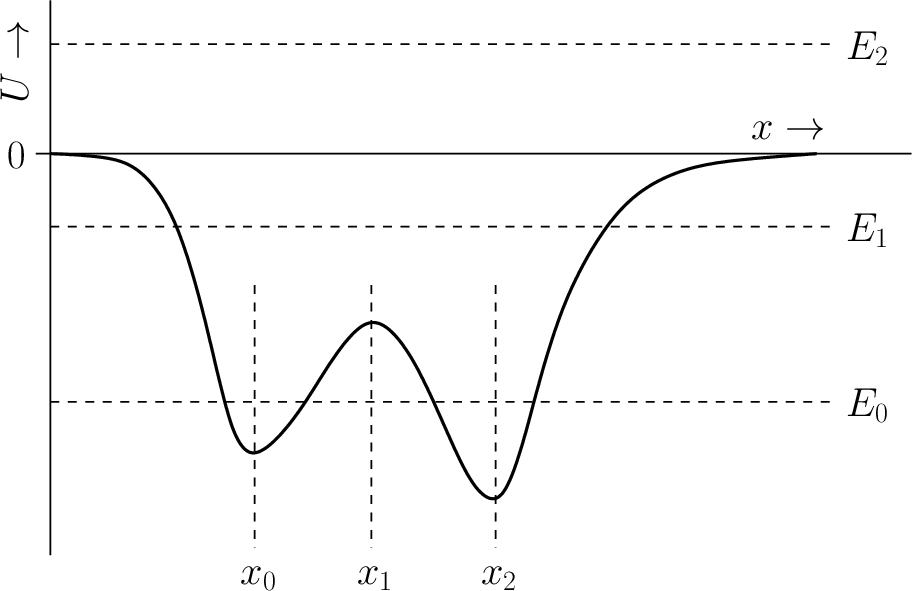, and.

Let us now make a distinction between stable equilibrium points and unstable equilibrium points. When the particle is slightly displaced from a stable equilibrium point then the resultant force acting on it must always be such as to return it to this point. In other words, ifis an equilibrium point then we require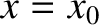(2.48)

for stability; that is, if the particle is displaced to the right, so that, then the force must act to the left, so that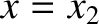, and vice versa. Likewise, if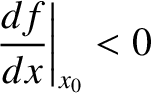(2.49)

then the equilibrium pointis unstable. It follows, from Equation (2.45), that stable equilibrium points are characterized by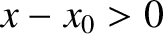(2.50)

In other words, a stable equilibrium point corresponds to a minimum of the potential energy curve,. Likewise, an unstable equilibrium point corresponds to a maximum of thecurve. Hence, we conclude that, in Figure 2.4,andare stable equilibrium points, whereasis an unstable equilibrium point. Of course, this makes perfect sense if we think ofas a gravitational potential energy curve, so thatis directly proportional to height. In this case, all we are saying is that it is easy to confine a low energy mass at the bottom of a smooth valley, but it is very difficult to balance the same mass on the top of a smooth hill (because any slight displacement of the mass will cause it to slide down the hill). Finally, if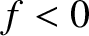(2.51)

at any point (or in any region) then we have what is known as a neutral equilibrium point. We can move the particle slightly away from such a point and it will still remain in equilibrium (i.e., it will neither attempt to return to its initial state, nor will it continue to move). A neutral equilibrium point corresponds to a flat spot in acurve. (See Figure 2.5.)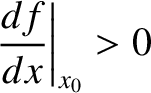The equation of motion of a particle moving in one dimension under the action of a conservative force is, in principle, integrable. Because, the energy conservation equation, Equation (2.46), can be rearranged to give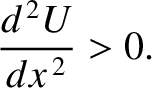(2.52)

where thesigns correspond to motion to the left and to the right, respectively. However, because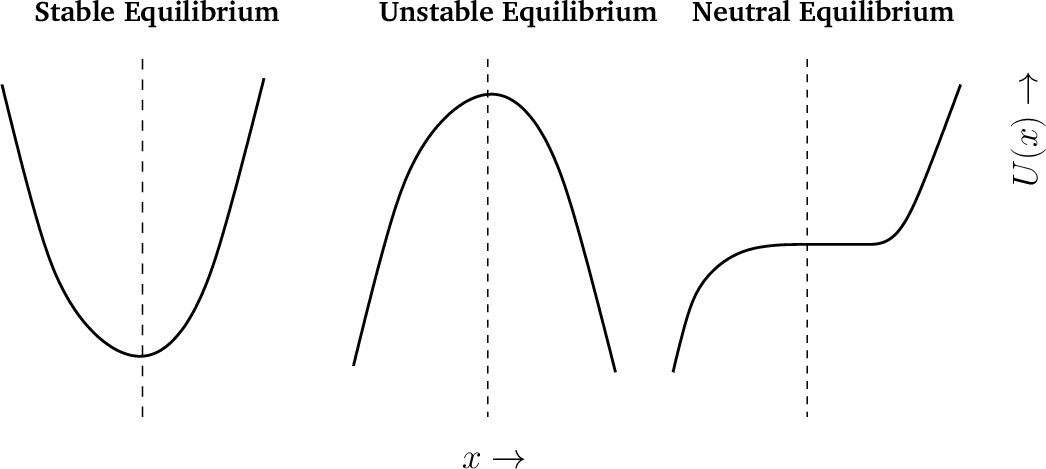, this expression can be integrated to give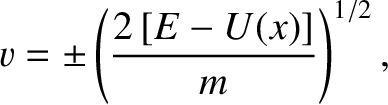(2.53)

where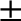. For sufficiently simple potential functions,, Equation (2.53) can be solved to giveas a function of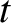. For instance, if,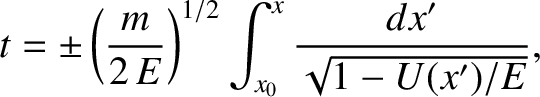, and the plus sign is chosen, then(2.54)

which can be inverted to give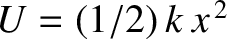(2.55)

where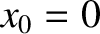and. Note that the particle reverses direction each time it reaches one of the so-called turning points (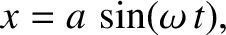) at which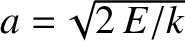(and, so,).Next: Simple harmonic motion Up: Newtonian mechanics Previous: Non-isolated systems
Richard Fitzpatrick 2016-03-31